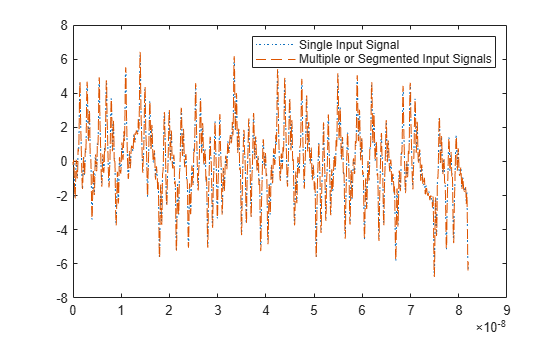# timeresp

Time response for rational objects

## Syntax

``[y,t,xf] = timeresp(h,inputsignal,ts)``
``[y,t,xf] = timeresp(h,inputsignal,ts,xo)``

## Description

example

````[y,t,xf] = timeresp(h,inputsignal,ts)` computes the time response `y`, sample time `t` of `y`, and final states of the system `xf`. The function calculates the time response for a `rational`, `rfmodel.rational`, or `rationalfit` object `h`, using the time-varying input signal `inputsignal` at the specified sample time `ts`.```
````[y,t,xf] = timeresp(h,inputsignal,ts,xo)` computes the time response using `xo` as the initial states of a system.```

## Examples

collapse all

Compute Time Response with Single Input Signal

Define an input signal.

```SampleTime = 2e-11; OverSamplingFactor = 25; TotalSampleNumber = 2^12; InputSignal = sign(randn(1, ... ceil(TotalSampleNumber/OverSamplingFactor))); InputSignal = repmat(InputSignal, [OverSamplingFactor, 1]); InputSignal = InputSignal(:);```

Create a `rational` function object.

```S = sparameters('default.s2p'); s21 = rfparam(S,2,1); datafreq = S.Frequencies; fit_data = rationalfit(datafreq,s21,'Tolerance',-32);```

Compute the time response with initial states set to `0`.

`[y,t,xf]=timeresp(fit_data,InputSignal,SampleTime);`

Compute Time Response with Segmented Input Signals

Segment the input signal into two parts.

Compute the time response of the first half of the input signal segment.

`[y2(1:2000,1),t21,xf2]=timeresp(fit_data,InputSignal(1:2000),SampleTime);`

Use the final states of the first half of the input signal segment `xf2` as the initial states to compute the time response of the second half of the input signal segment.

`[y2(2001:4100,1),t22,xf2]=timeresp(fit_data,InputSignal(2001:4100),SampleTime,xf2);`

Plot Time Responses

Plot the time responses of the single and segmented input signals.

```plot(t,y,':',t,y2,'--') legend({'Single Input Signal','Multiple or Segmented Input Signals'}) ```## Input Arguments

collapse all

Time-varying input signal, specified as a real finite vector.

Data Types: `double`

Rational function object, specified as a `rationalfit`, `rfmodel.rational`, or a `rational` object.

Data Types: `double`
Complex Number Support: Yes

Sample time of the input signal, specified as a positive scalar integer in seconds.

Data Types: `double`

Initial states of the system, specified as a real or complex column vector of length L, where L is equal to `length(h.ComplexPoles/2)+length(h.RealPoles)`.

Data Types: `double`

## Output Arguments

collapse all

Time-varying output signal, returned as a real finite vector.

Data Types: `double`

Sample time of the output signal, returned as a nonnegative vector of time values with discrete step size `ts` corresponding to the data in `y` in seconds.

Data Types: `double`

Final states of the system that the function uses in computing the last value of the `y`, returned as a real or complex column vector of length L, where L is equal to `length(h.ComplexPoles/2)+length(h.RealPoles)`.

Data Types: `double`

collapse all

### Output Signal Equation

`timeresp` function uses a variant of this equation to calculate the output signal.

`$Y\left(n\right)=sum\left(C.*X\left(n-Delay/ts\right)\right)+D*U\left(n-Delay/ts\right)$`

where

`$\begin{array}{l}X\left(n+1\right)=F*X\left(n\right)+G*U\left(n\right)\\ X\left(1\right)=0\\ F=\mathrm{exp}\left(A*ts\right)\\ G=\left(F-1\right)./A\end{array}$`

`A` — Complex vector of poles of the rational function

`C` — Complex vector of residues of the rational function

`D` — Scalar value specifying direct feedthrough

`Delay` — Delay time properties of the rational function object `h`. For more information, see `rationalfit` function.

## Version History

Introduced in R2007a

expand all